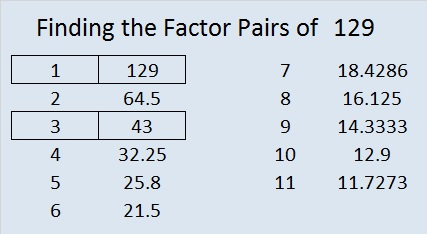# 129 and Level 2

129  is a composite number. 129 = 1 x 129 or 3 x 43. Factors of 129: 1, 3, 43, 129. Prime factorization: 129 = 3 x 43.2 + 3 + 5 + 7 + 11 + 13 + 17 + 19 + 23 + 29 = 129, thus 129 is the sum of the first 10 prime numbers.

129 is never a clue in the FIND THE FACTORS puzzles.Excel file of puzzles and previous week’s solutions: 10 Factors 2014-05-26This site uses Akismet to reduce spam. Learn how your comment data is processed.